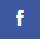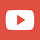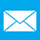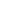# FAQ

What is a square meter?

As Square meter is an area of 1m x 1m

How do you calculate a square meter?

If you are measuring a square or rectangle area, multiply length times width; Length x Width = Area.
If it is a circular area you may need to use a formula.